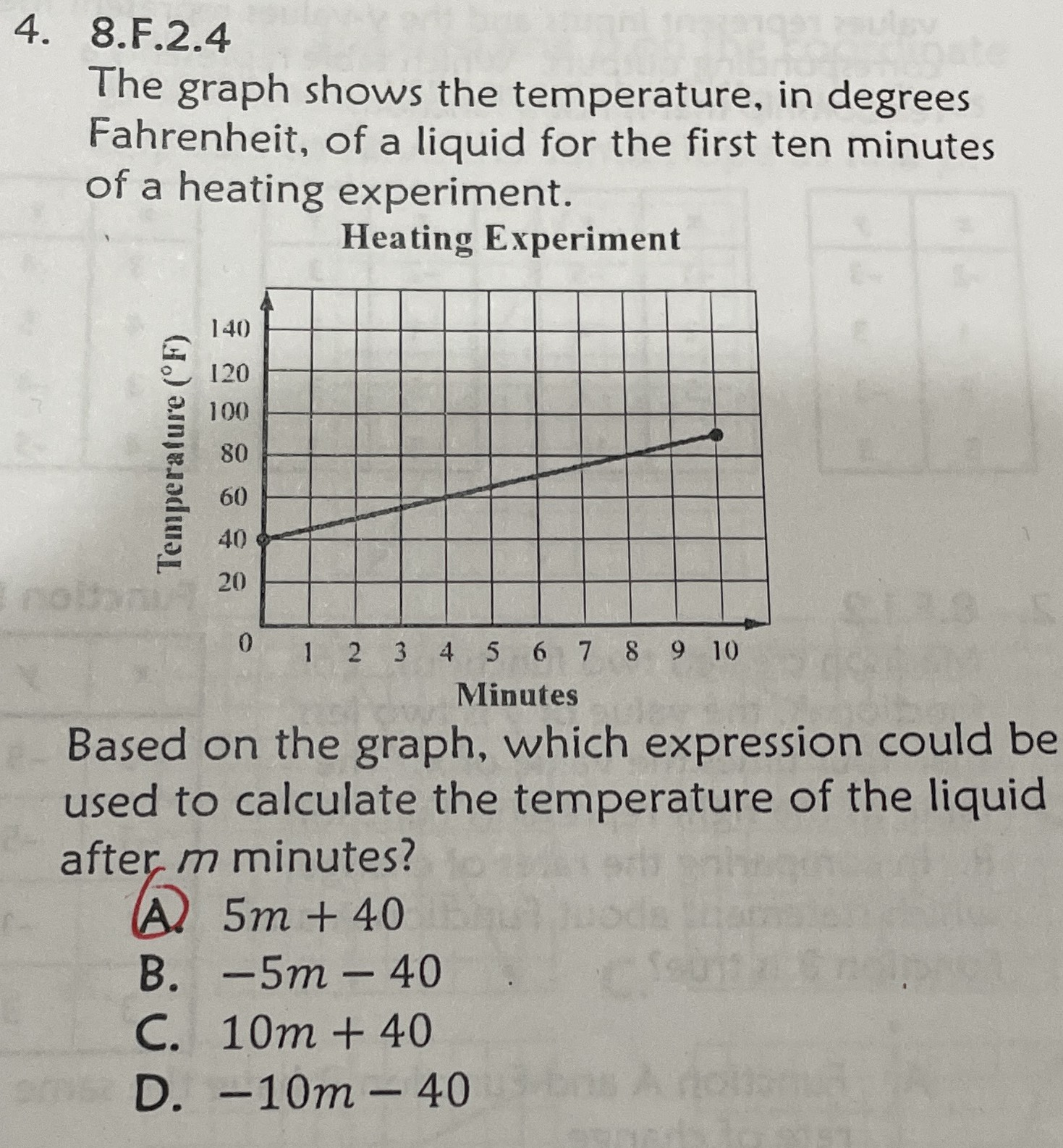### Still have math questions?

Algebra
QuestionThe graph shows the temperature, in degrees Fahrenheit, of a liquid for the first ten minutes of a heating experiment. Based on the graph, which expression could be used to calculate the temperature of the liquid after $$m$$ minutes?

A. $$5 m + 40$$

B. $$- 5 m - 40$$

C. $$10 m + 40$$

D. $$- 10 m - 40$$